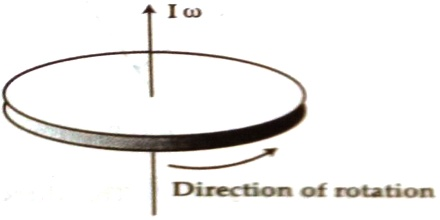Physics

# Vector form of Angular MomentumVector form of angular momentum: Angular momentum is a vector quantity. The direction of the vector is along the axis of rotation. If a right-hand screw is rotated along the direction of rotation of the particle then the direction in which the screw ad’ antes is the direction of the angular momentum vector [Figure].The angular momentum of an object is the product of its moment of inertia about the axis of rotation and its angular velocity. The direction of the angular-momentum vector is that of the axis of rotation of the given object and is designated as positive in the direction that a right-hand screw would advance if turned similarly. Appropriate MKS or SI units for angular momentum are kilogram metres squared per second (kg-m2/sec).